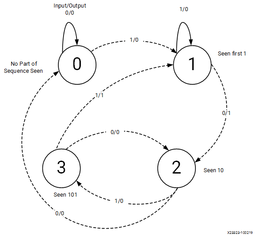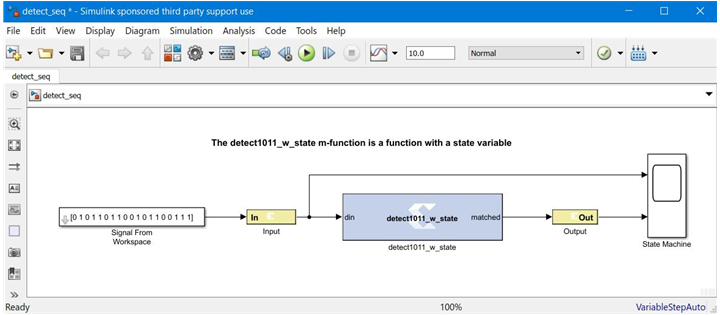# Finite State Machines - 2022.1 English

## Vitis Model Composer User Guide (UG1483)

Document ID
UG1483
Release Date
2022-05-26
Version
2022.1 English

This example shows how to create a finite state machine using the MCode block with internal state variables. The state machine illustrated below detects the pattern 1011 in an input stream of bits.

Figure 1. Finite State Machine DiagramThe M-function that is used by the MCode block contains a transition function, which computes the next state based on the current state and the current input. Unlike example 3 though, the M-function in this example defines persistent state variables to store the state of the finite state machine in the MCode block. The following M-code, which defines function `detect1011_w_state` is contained in file `detect1011_w_state.m`:

``````function matched = detect1011_w_state(din)
% This is the detect1011 function with states for detecting a
% pattern of 1011.

seen_none = 0; % initial state, if input is 1, switch to seen_1
seen_1 = 1;    % first 1 has been seen, if input is 0, switch
% seen_10
seen_10 = 2;   % 10 has been detected, if input is 1, switch to
% seen_1011
seen_101 = 3;  % now 101 is detected, is input is 1, 1011 is
% detected and the FSM switches to seen_1

% the state is a 2-bit register
persistent state, state = xl_state(seen_none, {xlUnsigned, 2, 0});

% the default value of matched is false
matched = false;

switch state
case seen_none
if din==1
state = seen_1;
else
state = seen_none;
end
case seen_1 % seen first 1
if din==1
state = seen_1;
else
state = seen_10;
end
case seen_10 % seen 10
if din==1
state = seen_101;
else
% no part of sequence seen, go to seen_none
state = seen_none;
end
case seen_101
if din==1
state = seen_1;
matched = true;
else
state = seen_10;
matched = false;
end
end
``````

The following diagram shows a state machine Subsystem containing a MCode block after compilation; the MCode block uses M-function `detect1101_w_state`.

Figure 2. Subsystem Containing MCode Block After Compilation